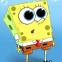Java Games: Flashcards, matching, concentration, and word search.# Activity #1: Properties Match-Up

In this activity you will do one of two things. You will either match the addition and multiplication properties with the definitions or examples of the properties, or you will match equivalent expressions that demonstrate the distributive property.

In this activity, the / symbol is used to signify a fraction.

AB
Commutitive Property of Addition-12 + 18 = 18 + (-12)
Commutative Property of Multiplication5(-9)(2) = 5(2)(-9)
Associative Property of Addition5 +(15 + 18) = (5 + 15) +18
Associative Property of Multiplication[(-6)(8)](5) = (-6)[(8)(5)]
Inverse Property of Addition121 + (-121) = 0
Inverse Property of Multiplication16(1/16) = 1
Identity Property of Addition(-5/8) + 0 = -5/8
Identity Property of Multiplication1(36) = 36
Additive Inversethe opposite of the integer
Multiplicative Inversethe reciprocal (flip) of the numberThis activity was created by a Quia Web subscriber. Learn more about QuiaCreate your own activities